# Catalan numbers

The Catalan numbers: 1, 2, 5, 14, 42, 132, 429, 1430, 4862, 16796, 58786, 208012, 742900, 2674440, 9694845, ..., named after Eugéne Charles Catalan (1814--1894), arise in a number of problems in combinatorics.

They can be computed using the formula: $\large\frac 1 {n+1}{_{2n}C_n}$

and satisfy the recurrence relation $C_{0} =1$, $C_{n+1} = \large\frac{2(2n+1)}{n+2}\normalsize C_{n}$

Among other things, the Catalan numbers describe

• Polygons: the number of ways a polygon with $n+2$ sides can be cut into $n$ triangles.
• Parentheses: the number of ways in which parentheses can be placed in a sequence of numbers to be multiplied, two at a time.
• Trees: the number of rooted, trivalent trees with $n+1$ nodes.
• Paths: the number of paths of length $2n$ through an $n \times n$ grid that do not rise above the main diagonal,
• Stairs: the number of ways to decompose a staircase-shaped figure with $n$ steps into $n$ rectangles.

#### Polygons

4 sides, 2 ways: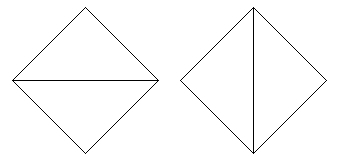5 sides, 5 ways: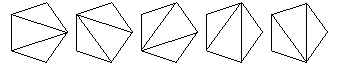6 sides, 14 ways:7 sides, 42 ways:8 sides, 132 ways: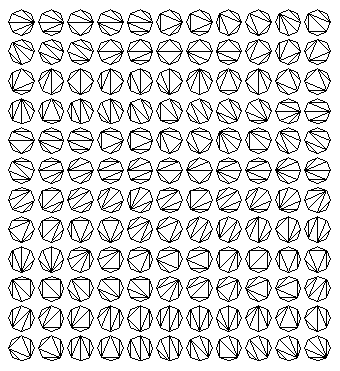9 sides, 429 ways:#### Multiplication diagrams

3 numbers:

(1 (2 3))   ((1 2) 3)

4 numbers:

(1 (2 (3 4)))   (1 ((2 3) 4))
((1 2) (3 4))   ((1 (2 3)) 4)
(((1 2) 3) 4)


5 numbers:

(1 (2 (3 (4 5))))   (1 (2 ((3 4) 5)))
(1 ((2 3) (4 5)))   (1 ((2 (3 4)) 5))
(1 (((2 3) 4) 5))   ((1 2) (3 (4 5)))
((1 2) ((3 4) 5))   ((1 (2 3)) (4 5))
((1 (2 (3 4))) 5)   ((1 ((2 3) 4)) 5)
(((1 2) 3) (4 5))   (((1 2) (3 4)) 5)
(((1 (2 3)) 4) 5)   ((((1 2) 3) 4) 5)


6 numbers:

(1 (2 (3 (4 (5 6)))))   (1 (2 (3 ((4 5) 6))))
(1 (2 ((3 4) (5 6))))   (1 (2 ((3 (4 5)) 6)))
(1 (2 (((3 4) 5) 6)))   (1 ((2 3) (4 (5 6))))
(1 ((2 3) ((4 5) 6)))   (1 ((2 (3 4)) (5 6)))
(1 ((2 (3 (4 5))) 6))   (1 ((2 ((3 4) 5)) 6))
(1 (((2 3) 4) (5 6)))   (1 (((2 3) (4 5)) 6))
(1 (((2 (3 4)) 5) 6))   (1 ((((2 3) 4) 5) 6))
((1 2) (3 (4 (5 6))))   ((1 2) (3 ((4 5) 6)))
((1 2) ((3 4) (5 6)))   ((1 2) ((3 (4 5)) 6))
((1 2) (((3 4) 5) 6))   ((1 (2 3)) (4 (5 6)))
((1 (2 3)) ((4 5) 6))   ((1 (2 (3 4))) (5 6))
((1 (2 (3 (4 5)))) 6)   ((1 (2 ((3 4) 5))) 6)
((1 ((2 3) 4)) (5 6))   ((1 ((2 3) (4 5))) 6)
((1 ((2 (3 4)) 5)) 6)   ((1 (((2 3) 4) 5)) 6)
(((1 2) 3) (4 (5 6)))   (((1 2) 3) ((4 5) 6))
(((1 2) (3 4)) (5 6))   (((1 2) (3 (4 5))) 6)
(((1 2) ((3 4) 5)) 6)   (((1 (2 3)) 4) (5 6))
(((1 (2 3)) (4 5)) 6)   (((1 (2 (3 4))) 5) 6)
(((1 ((2 3) 4)) 5) 6)   ((((1 2) 3) 4) (5 6))
((((1 2) 3) (4 5)) 6)   ((((1 2) (3 4)) 5) 6)
((((1 (2 3)) 4) 5) 6)   (((((1 2) 3) 4) 5) 6)


#### Tree diagrams:

3 nodes: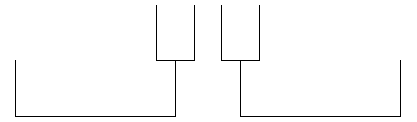4 nodes:5 nodes: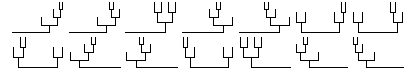6 nodes: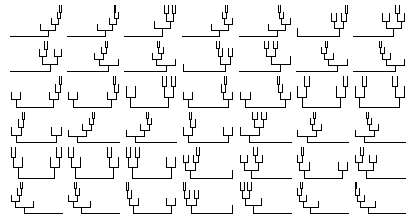7 nodes: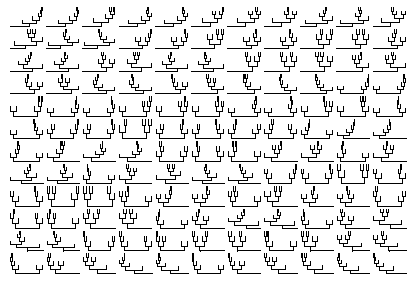8 nodes: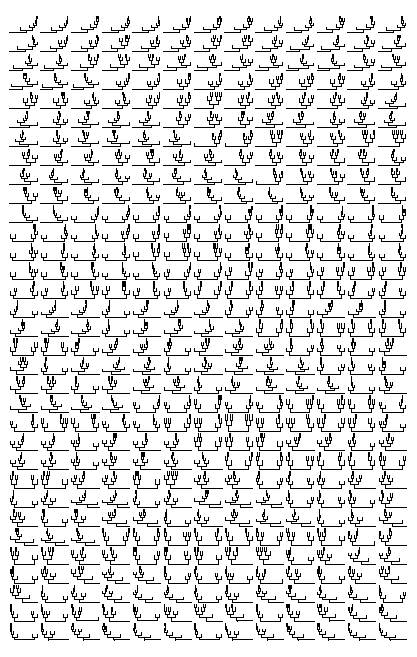#### Path diagrams:

2 x 2 grid: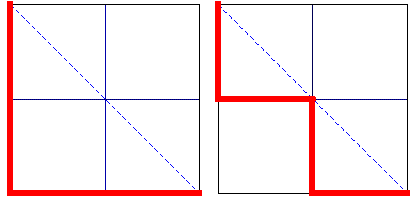3 x 3 grid: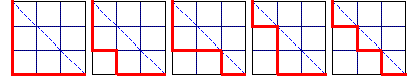4 x 4 grid:5 x 5 grid: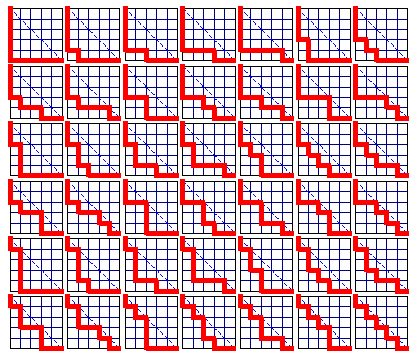6 x 6 grid: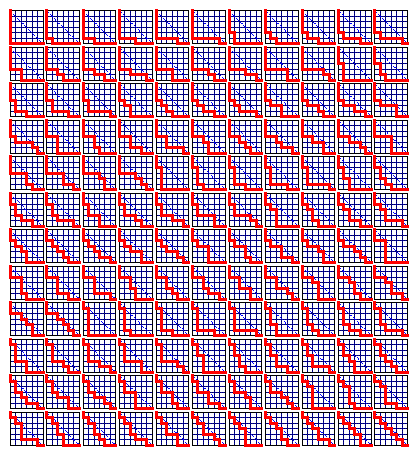#### Staircases:

$n$ = 2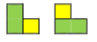$n$ = 3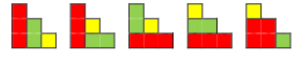$n$ = 4$n$ = 5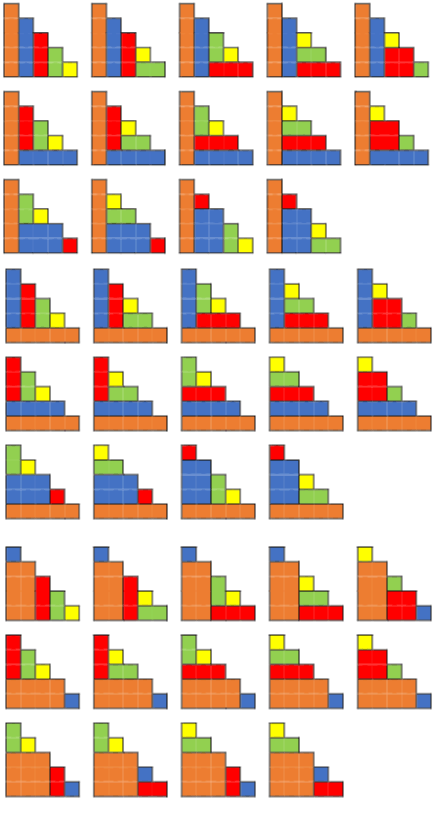Robert M Dickau, Heinz Klaus Strick

### References (show)

1. B Hayes, A Question of Numbers, American Scientist, January-February 1996
2. S S Skiena, Implementing Discrete Mathematics: Combinatorics and Graph Theory with Mathematica, Addison-Wesley, 1990
3. F S Roberts, Applied Combinatorics, Prentice-Hall, 1984
4. D E Knuth, Sorting and Searching (vol. 3 of The Art of Computer Programming), Addison-Wesley,
5. Martin Gardner, Time Travel and Other Mathematical Bewilderments, chapter 20, W. H. Freeman, 1988.
6. Ilan Vardi, Computational Recreations in Mathematica, chapter 9, Addison-Wesley, 1991.